Question

# Suppose that a firm had a production function given by: q=L^0.25*K^0.75. The wage rate (w) is...

Suppose that a firm had a production function given by: q=L^0.25*K^0.75. The wage rate (w) is \$10 and the rental rate (r) is \$20. Calculate the amount of labor the firm would hire when it produces 300 units of output in a cost-minimizing way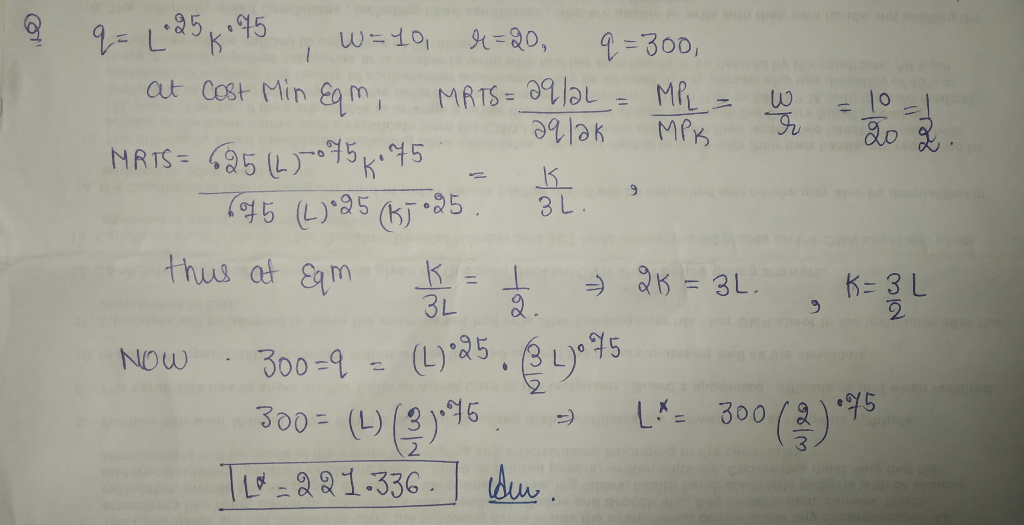#### Earn Coins

Coins can be redeemed for fabulous gifts.

Similar Homework Help Questions
• ### Suppose that a firm had a production function given by q-21 K The rental rate for...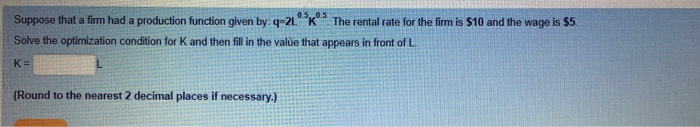Suppose that a firm had a production function given by q-21 K The rental rate for the fim is \$10 and the wage is s5 Solve the optimization condition for K and then fill in the value that appears in front of L K- Round to the nearest 2 decimal places if necessary.) Suppose that a firm had a production function given by q-L025k075. The wage rate (w) is \$10 and the rental rate () is \$10 Calculate the amount...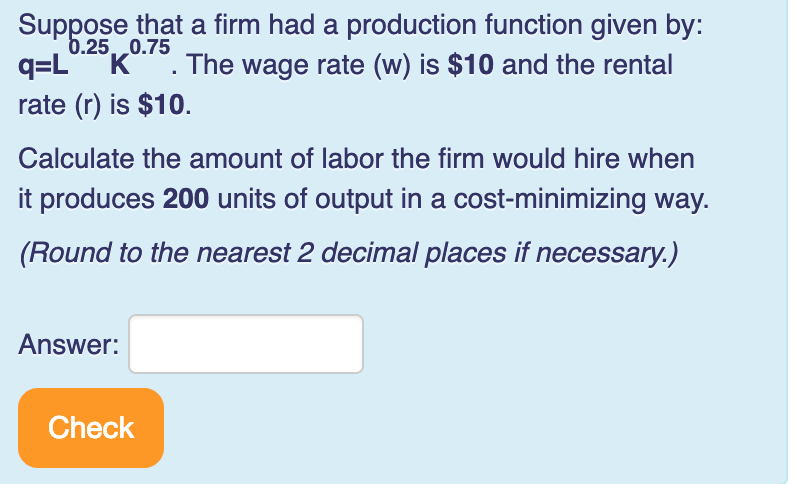Please help me! Thank you! Suppose that a firm had a production function given by: q-L K. The wage rate (w) is \$10 and the rental rate (r) is \$10 Calculate the amount of labor the firm would hire when t produces 200 units of output in a cost-minimizing way. .25, 0.75 (Round to the nearest 2 decimal places if necessary.) Answer: Check

• ### Suppose that a firm had a production function given by: q=L025K0 75 The wage rate (...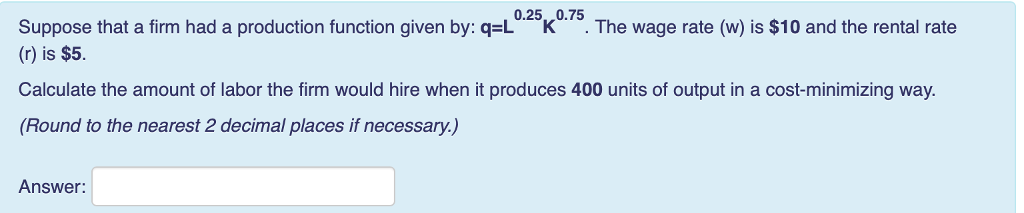Suppose that a firm had a production function given by: q=L025K0 75 The wage rate ( \$10 and the rental rate (r) is \$5 Calculate the amount of labor the firm would hire when it produces 400 units of output in a cost-minimizing way (Round to the nearest 2 decimal places if necessary.) Answer:

• ### Question 10 Tries remaining: 2 Suppose that a firm had a production function given by: q=Lºkº....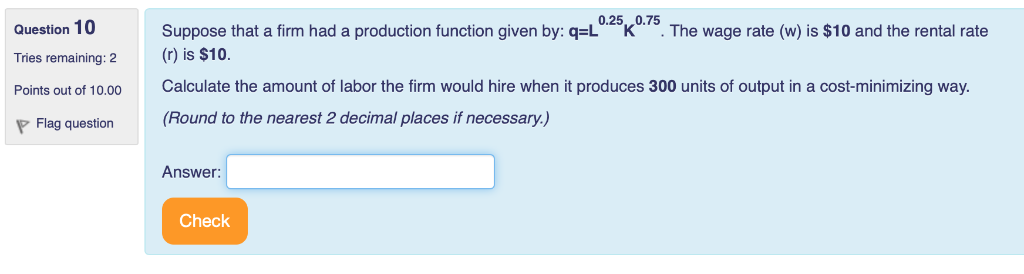Question 10 Tries remaining: 2 Suppose that a firm had a production function given by: q=Lºkº. The wage rate (w) is \$10 and the rental rate () is \$10. Calculate the amount of labor the firm would hire when it produces 300 units of output in a cost-minimizing way. (Round to the nearest 2 decimal places if necessary.) Points out of 10.00 P Flag question Answer: Check

• ### A firm has a Cobb-Douglas production function of Q = K^(0.25) L^(0.75) (a) Does this production...

A firm has a Cobb-Douglas production function of Q = K^(0.25) L^(0.75) (a) Does this production technology exhibit increasing, constant, or decreasing returns to scale? (b) Suppose that the rental rate of capital is r = 1, the wage rate is w = 1, and the ?rm wants to produce Q = 3. In the long-run, what combination of L and K should they use? (It would be good to practice doing this with the Lagrangian, even if you can...

• ### A firm’s production technology is given by the production function q = 0.25 LK where L...

A firm’s production technology is given by the production function q = 0.25 LK where L represents labor hours, K machine hours and q the amount of output. The market wage and rental rates are, w= \$16 and r = \$256. The firm is operating in the long run where it can adjust both inputs. (a) Suppose that the firm currently is using ten labor hours for each machine hour. Is it minimizing its long run total cost? If so...

• ### Suppose the production function for a firm is given by: q=4L^0.75*K^0.25. If the firm currently has...

Suppose the production function for a firm is given by: q=4L^0.75*K^0.25. If the firm currently has 20 units of capital (K) and 10 units of labor (L), then calculate the Marginal Rate of Technical Substitution (MRTSLK).

• ### A firm’s production technology is given by the production function q  0.25 LK where L...

A firm’s production technology is given by the production function q  0.25 LK where L represents labor hours, K machine hours and q the amount of output. The market wage and rental rates are, w= \$16 and r = \$256. The firm is operating in the long run where it can adjust both inputs. Suppose that the firm currently is using ten labor hours for each machine hour. Is it minimizing its long run total cost? If so why...

• ### Question 9 0.5,,0.25 Suppose that a firm had a production function given by: q-2L K ....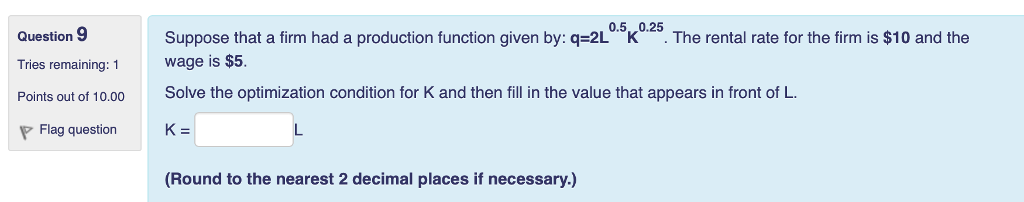Question 9 0.5,,0.25 Suppose that a firm had a production function given by: q-2L K . The rental rate for the firm is \$10 and the Wage is \$5. Solve the optimization condition for K and then fill in the value that appears in front of L. Tries remaining: 1 Flag question K (Round to the nearest 2 decimal places if necessary.)

• ### Suppose that a firm has a production function given by: ? = ?^?.???^?.? . The wage...

Suppose that a firm has a production function given by: ? = ?^?.???^?.? . The wage rate is \$18 and the rental rate is \$9. 12. Suppose that the firm has 4 units of capital in the short run. Find the short run total cost function. ________________________________ 13. Find the marginal product of labor (MPL) function. ________________________________ 14. Solve the optimization condition for K and write that equation. ________________________________ 15. Suppose the firm wants to minimize the cost of producing...